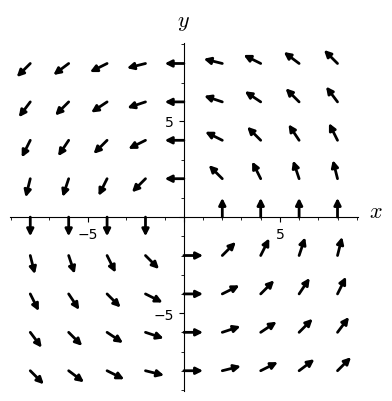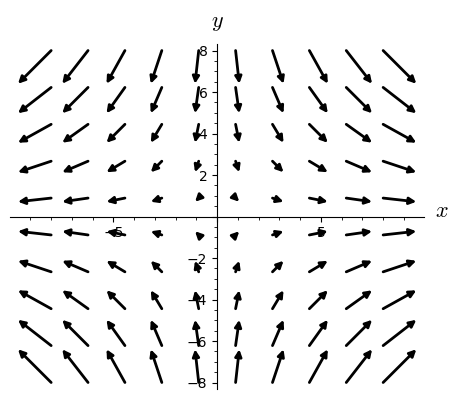# 11. Vector fields¶

This notebook is part of the Introduction to manifolds in SageMath by Andrzej Chrzeszczyk (Jan Kochanowski University of Kielce, Poland).

In :
version()

Out:
'SageMath version 9.6, Release Date: 2022-05-15'

A vector field $X$ on a manifold $M$ assigns to a point $p ∈ M$ a tangent vector $X(p) ∈ T_p M$. Instead of $X(p)$ we shall write $X_p$. Since a vector field gives us a tangent vector at each point of its domain and a tangent vector can be applied to real-valued smooth functions to yield real numbers, given a vector field $X$ and $f ∈ C^∞ (M)$, we can form a real-valued function $X(f)=Xf$, defined by \begin{equation} (X f )( p) ≡ X_p( f ). \label{}\tag{11.1} \end{equation}

We have also an equivalent definition
A vector field $X$ on a manifold $M$ is a linear map $X : C^∞ (M ) → C^∞ (M )$ such that

$$X(f g) = f X(g) + gX(f )\quad \mbox{ for all } f, g ∈ C^∞ (M ).$$

A vector field $X$ is smooth if for all $f\in C^\infty(M)$ the function $Xf$ is also in $C^\infty(M)$.
Equivalently we can say that a vector field $X$ on a manifold $M$ is smooth if for any coordinate chart $φ = (x^1 ,\ldots , x^n ) :U → R^n$ we have for any point $p ∈ U$ \begin{equation} X(p) = f^i(p)\frac{\partial}{\partial x^i}\Big|_p \label{}\tag{11.2} \end{equation} for some $C^\infty$ functions $f^i : U → R$.

### Frames¶

To define vector fields with specified components in SageMath Manifolds we need vector frames.

If $U$ is an open subset of a manifold $M$ (for example a coordinate neighborhood) then the vector frame on $U$ is the sequence $e$ of vector fields on $U$ such that for each $p∈U, \ e(p)$ is a vector basis of the tangent space $T_pU$.

Usually we shall use the coordinate frames associated with the local coordinates.

Example 11.1

Let us display the frame corresponding to the default chart

In :
%display latex
R2=Manifold(2,'R^2',start_index=1)     # manifold R^2
c_xy.<x,y>=R2.chart()                  # global coordinates
v = R2.vector_field(name='v')          # vector field on R2
e = c_xy.frame()                       # vector frame
print(e)
e

Coordinate frame (R^2, (∂/∂x,∂/∂y))

Out:
$\displaystyle \left(R^2, \left(\frac{\partial}{\partial x },\frac{\partial}{\partial y }\right)\right)$

In this case the elements of the frame are the vector fields $p\to \frac{\partial}{\partial x^i}\big|_p\quad$ ($\frac{\partial}{\partial x^i}\big|_p$ was defined by formula (8.3)).

If the frame is not defined by the user, the default frame is used.

Let us check how the elements e,e of our frame e act on a scalar function.

In :
# continuation                   # f is a scalar function on R2:
f = R2.scalar_field(function('f')(x,y), name='f')
e(f).expr(), e(f).expr()   # values of the vector frame on f

Out:
$\displaystyle \left(\frac{\partial}{\partial x}f\left(x, y\right), \frac{\partial}{\partial y}f\left(x, y\right)\right)$

If the frame is defined, we can define the components of the vector field in this frame.

Example 11.2

Here defining a vector field, we use frame e:

In :
R2=Manifold(2,'R^2',start_index=1)     # manifold R2
c_xy.<x,y>=R2.chart()                  # global coordinates
v = R2.vector_field(name='v')          # vector field v
e = c_xy.frame()                       # vector frame e
v[e,:] = [-y, 1+x]                     # components of v in e
v.display()                            # show v

Out:
$\displaystyle v = -y \frac{\partial}{\partial x } + \left( x + 1 \right) \frac{\partial}{\partial y }$

Since we use the default frame, the previous code can be simplified.

Example 11.3

Here the frame is not specified, the result is the same, since we have used the default frame

In :
R2=Manifold(2,'R^2',start_index=1)     # manifold R2
c_xy.<x,y>=R2.chart()                  # global coordinates
v = R2.vector_field(name='v')          # vector field
v[:] = [-y, 1+x]               # components of v in default frame
v.display()                            # show v

Out:
$\displaystyle v = -y \frac{\partial}{\partial x } + \left( x + 1 \right) \frac{\partial}{\partial y }$

The value of v on a scalar function:

In :
# continuation                         # scalar function
f = R2.scalar_field(function('f')(x,y), name='f')
s=v(f)                                 # value of v on f
s.expr()                               # show expression

Out:
$\displaystyle -y \frac{\partial}{\partial x}f\left(x, y\right) + {\left(x + 1\right)} \frac{\partial}{\partial y}f\left(x, y\right)$

Example 11.4

Let us check that vector fields are derivations.

In :
# vector fields are derivations
R2=Manifold(2,'R^2',start_index=1)        # manifold R2
c_xy.<x,y>=R2.chart()                     # global coordinates
v = R2.vector_field(name='v')             # vector field v
e = c_xy.frame()                          # frame on R2
f=R2.scalar_field(function('f')(x,y))     # scalar function f
g=R2.scalar_field(function('g')(x,y))     # scalar function g
v1=R2.scalar_field(function('v1')(x,y))   # component v1 of v
v2=R2.scalar_field(function('v2')(x,y))   # component v2 of v
v[e,:] = [v1, v2]                         # components of v in frame e


First we compute the value of $\ v(fg)$:

In :
v(f*g).expr()                             # value of v on f*g

Out:
$\displaystyle g\left(x, y\right) v_{1}\left(x, y\right) \frac{\partial}{\partial x}f\left(x, y\right) + g\left(x, y\right) v_{2}\left(x, y\right) \frac{\partial}{\partial y}f\left(x, y\right) + f\left(x, y\right) v_{1}\left(x, y\right) \frac{\partial}{\partial x}g\left(x, y\right) + f\left(x, y\right) v_{2}\left(x, y\right) \frac{\partial}{\partial y}g\left(x, y\right)$

and next the value of $\ fv(g)+gv(f)$:

In :
(f*v(g)+g*v(f)).expr()                    #  value of f*v(g)+g*v(f)

Out:
$\displaystyle g\left(x, y\right) v_{1}\left(x, y\right) \frac{\partial}{\partial x}f\left(x, y\right) + g\left(x, y\right) v_{2}\left(x, y\right) \frac{\partial}{\partial y}f\left(x, y\right) + f\left(x, y\right) v_{1}\left(x, y\right) \frac{\partial}{\partial x}g\left(x, y\right) + f\left(x, y\right) v_{2}\left(x, y\right) \frac{\partial}{\partial y}g\left(x, y\right)$

Both values are the same:

In :
v(f*g) == f*v(g) + g*v(f)                 # check if v is derivation

Out:
$\displaystyle \mathrm{True}$

Example 11.5

Let us plot the vector field $\quad v_0=\frac{-y}{\sqrt{x^2+y^2}}\frac{\partial}{\partial x} +\frac{x}{\sqrt{x^2+y^2}}\frac{\partial}{\partial y}.$

To obtain the plot, some restriction are necessary.

In :
%display latex
R2=Manifold(2,'R^2',start_index=1)        # manifold R2
c_xy.<x,y>=R2.chart(coord_restrictions=lambda x,y:x^2+y^2>0)
v0=R2.vector_field(name='v0')             # vector field v0
v0[:]=-y/sqrt(x^2+y^2),x/sqrt(x^2+y^2)    # components of v0
v0.display()

Out:
$\displaystyle v0 = \left( -\frac{y}{\sqrt{x^{2} + y^{2}}} \right) \frac{\partial}{\partial x } + \left( \frac{x}{\sqrt{x^{2} + y^{2}}} \right) \frac{\partial}{\partial y }$
In :
v0.plot(arrowsize=2,color='black',aspect_ratio=1)  # plot v0

Out:Use the command v0.plot? to see how to plot vector fields.

Example 11.6

Now let us plot the vector field $\quad v_1=x\frac{\partial}{\partial x} -y\frac{\partial}{\partial y}.$

Here we have excluded some neighborhood of the origin.

In :
M=Manifold(2,'M')               # manifold M
X.<x,y>=M.chart(coord_restrictions=lambda x,y:(abs(x)>0.1,abs(y)>0.1))
e=X.frame()                     # frame e on M
v1=M.vector_field(name='v1')    # vector field v1
v1[e,:]=x,-y                    # components of v1
v1.plot(number_values=10, scale=0.2,
arrowsize=2,color='black',aspect_ratio=1)  # plot v1

Out:Example 11.7

Let us consider the two-dimensional sphere with spherical coordinates $\theta,\phi$ and the corresponding frame $\frac{\partial}{\partial\theta},\frac{\partial}{\partial\phi}$.

In :
%display latex
S2=manifolds.Sphere(2)                    # sphere S^2
Phi=S2.embedding()                        # embedding S^2 -> E^3
sph.<th,ph>=S2.spherical_coordinates()    # spherical coord. on S^2
sphFr=sph.frame()                         # frame on S^2
E=S2.ambient()                            # Euclidean space E^3
cart.<x,y,z> = E.cartesian_coordinates()  # Cart.coord. in E^3


First let us plot the vector field $\frac{\partial}{\partial\phi}$:

In :
p1=sphFr.plot(chart=cart, mapping=Phi,number_values=10,
scale=0.5,arrowsize=0.5,label_axes=False,thickness=0.5,
width=0.5,color='black')          # plot vector field d/dphi
s=sphere(color='lightgrey',opacity=0.9)   # plot the sphere
(p1+s).show(frame=False,label_axes=False) # combine plots


and next $\frac{\partial}{\partial\theta}$:

In :
p3=sphFr.plot(chart=cart, mapping=Phi,number_values=10,
scale=0.3,arrowsize=0.5,label_axes=False,thickness=0.5,
width=0.5,color='black')      # plot vector field d/dtheta
(s+p3).show(frame=False,label_axes=False)  # combine plots


### Vector fields of tangents to a curve¶

We can also define a vector fields of tangents to a curve $\gamma$ in a manifold $M$ i.e. the map $t\to \gamma'(t)\in T_{\gamma(t)}M$ defined for $t$ from some interval $I$.

Example 11.8

Let us plot the vector field of tangent vectors to the curve $\ \gamma\$ defined in Cartesian coordinates by $(x(t),y(t),z(t))=(\cos(3t),\sin(3t),2t)$.

In :
R.<t> = manifolds.RealLine()         # Real line with coordinate t
R3 = Manifold(3, 'R^3')              # manifold R3
X.<x,y,z> = R3.chart()               # cartesian coordinates in R3
# curve c definition
c=R3.curve({X:[cos(3*t),sin(3*t),2*t]},(t, -pi/2, +pi/2),name='c')
# plot curve:
p=c.plot(X,color='grey',thickness=3,plot_points=200,label_axes=False)
vc = c.tangent_vector_field()        # vector field vc along c
p2=vc.plot(chart=X, number_values=40, scale=0.15,
arrowsize=0.5,thickness=0.5,width=0.5,
color='black', label_axes=False)   # plot the vector field
(p+p2).rotateZ(pi/2).show(figsize=[4,3],aspect_ratio=[1,1,0.4],
label_axes=False)                  # combine plots


Example 11.9

Now let us try to plot a curve on the sphere and the vector field of tangents to that curve.

We start from preparation commands from Example 11.7.

In :
S2=manifolds.Sphere(2)                    # sphere S^2
Phi=S2.embedding()                        # embedding S^2 -> E^3
sph.<th,ph>=S2.spherical_coordinates()    # spherical coord.on S^2
sphFr=sph.frame()                         # frame on S^2
E=S2.ambient()                            # Euclidean space E^3
cart.<x,y,z> = E.cartesian_coordinates()  # Cartesian coord.in E^3


Next we define the curve and the vector field of tangents.

In :
R.<t> = manifolds.RealLine()              # real line
c = S2.curve({sph: [t, cos(2*t)]},        # curve th=t,ph=cos(2t)
(t, -oo, +oo), name='c')
vc = c.tangent_vector_field()             # vector field of tangents


Now we plot the curve:

In :
p4=c.plot(chart=cart,mapping=Phi,thickness=2,color='black',
plot_points=200, aspect_ratio=1,label_axes=False)  # plot curve


the vector field of tangents:

In :
p5=vc.plot(chart=cart,mapping=Phi, number_values=40, scale=0.2,
arrowsize=0.3,thickness=0.3,width=0.3,color='black',
label_axes=False)                      # plot vector field


and the sphere:

In :
s=sphere(color='lightgrey',opacity=0.6)  # plot sphere


Finally we combine the three plots.

In :
(s+p4+p5).rotateZ(pi/2).show(frame=False,label_axes=False) # combine plots